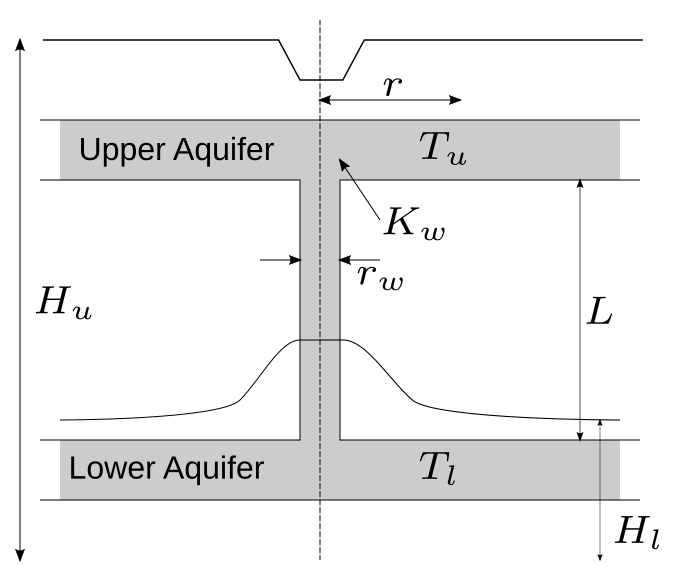# Borehole Function

The 8-dimensional borehole function models water flow through a borehole that is drilled from the ground surface through the two aquifers. The water flow rate is described by the borehole and the aquifer’s properties. The borehole function is typically used to benchmark metamodeling and sensitivity analysis methods (Harper and Gupta, 1983; Morris, 1993; An and Owen, 2001; Kersaudy et al., 2015).

## Description

The water flow rate through the borehole (Q) is computed using the following analytical expression:

Q = f(\mathbf{x}) = \frac{2\pi T_u (H_u - H_l)}{\ln{(r/r_w)} \left[1 + \frac{2 L T_u}{\ln{(r/rw)} r_w^2 K_w} + \frac{T_u}{T_l}\right]},

where \mathbf{x} = \{r_w, r, T_u, H_u, T_l, H_l, L, K_w \}​ is the vector of input variables defined below. The configuration of the scenario of the water flow is illustrated in Figure 1.Figure 1: Illustration for the water flow through the borehole, adapted from Harper and Gupta (1983).

## Inputs

For metamodeling applications (Morris et al., 1993; An and Owen, 2001; Kersaudy et al., 2015), the eight input variables of the borehole function are modeled as independent uniform random variables with specified ranges shown in the table below.

No Variable Distribution Parameters Description
1 r_w Uniform r_{w,\min} = 0.05,
r_{w,\max} = 0.15
2 r Uniform r_{\min} = 100,
r_{\max} = 50'000
3 T_u Uniform T_{u,\min} = 63'070,
T_{u,\max} = 115'600
Transmissivity of upper aquifer [\text{m}^2/\text{year}]
4 H_u Uniform H_{u,\min} = 990,
H_{u,\max} = 1'100
Potentiometric head of upper aquifer [\text{m}]
5 T_l Uniform T_{l,\min} = 63.1,
T_{l,\max} = 116
Transmissivity of lower aquifer [\text{m}^2/\text{year}]
6 H_l Uniform H_{l,\min} = 700,
H_{l,\max} = 820
Potentiometric head of lower aquifer [\text{m}]
7 L Uniform L_{\min} = 1'120,
L_{\max} = 1'680
Length of borehole [\text{m}]
8 K_w Uniform K_{w,\min} = 9'885,
K_{w,\max} = 12'045
Hydraulic conductivity of borehole [\text{m}/\text{year}]

Other literature (e.g., Harper and Gupta, 1983) put alternative distributions on the variables r_w and r (while keeping the others the same) as shown in the table below.

No Variable Distribution Parameters Description
1 r_w Gaussian \mu_{r_w} = 0.10,
\sigma^2_{r_w} = 0.0161812
2 r Lognormal \lambda_r = 7.71,
\xi_r = 1.0056

## Resources

The vectorized implementation of the borehole function in MATLAB as well as the script file with the model and probabilistic inputs definitions for the function in UQLAB can be downloaded below:

uq_borehole.zip (3.1 KB)

The contents of the file are:

Filename Description
uq_borehole.m vectorized implementation of the borehole function in MATLAB
uq_Example_borehole.m definitions for the model and probabilistic inputs in UQLab
LICENSE license for the function (BSD 3-Clause)

## References

• W. V. Harper and S. K. Gupta, “Sensitivity/Uncertainty Analysis of a Borehole Scenario Comparing Latin Hypercube Sampling and Deterministic Sensitivity Approaches”, Office of Nuclear Waste Isolation, Battelle Memorial Institute, Columbus, Ohio, BMI/ONWI-516, 1983. URL
• M. D. Morris, T. J. Mitchell, and D. Ylvisaker, “Bayesian design and analysis of computer experiments: Use of derivatives in surface prediction,” Technometrics, vol. 35, no. 3, pp. 243–255, 1993. DOI:10.1080/00401706.1993.10485320
• J. An and A. Owen, “Quasi-regression,” Journal of Complexity, vol. 17, pp. 588–607, 2001. DOI:10.1006/jcom.2001.0588
• P. Kersaudy, B. Sudret, N. Varsier, O. Picon, and J. Wiart, “A new surrogate modeling technique combining Kriging and polynomial chaos expansions – Application to uncertainty analysis in computational dosimetry,” Journal of Computational Physics, vol. 286, pp. 103–117, 2015. DOI:10.1016/j.jcp.2015.01.034